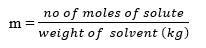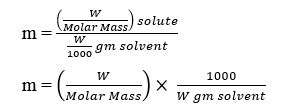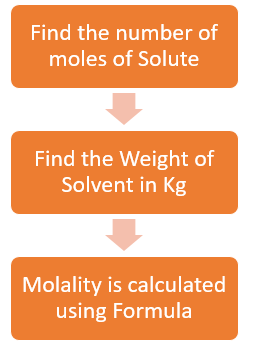# Molality Formula

Table of Content

## Molatity Definition & Formula

• Molality is also known as Molal Concentration and It is one measure for Solute concentration
• Molality is defined as the number of Moles of solute dissolved per 1000 g (1 kg) of solvent.
• Molality is expressed as 'm'unit = Moles kg-1
• Molality Formula is given as• Let weight of solvent = 1 kg
m = n solute
• Molality Formula can also be expressed as## How to Calculate Molatity• Find the Number of moles of Solute. if Mass is given ,then find the Molar mass, then calculate the Moles
• Find the mass of the solvent in Kg. If volume and density is given, then mass can be calculated using $\text{Density}=\frac {\text{mass}}{\text{volume}}$
• Now that both moles and Solvent mass in kg is known, we can calculate the Molality using Molality Formula

## Difference Between Molarity and Molality

 Molarity Molality number of moles of solute per liter of solution. number of moles of solute per kilogram of solvent. Molarity is a measurement of the moles in the total volume of the solution, molality is a measurement of the moles in relationship to the mass of the solvent. Unit is Moles L-1 Unit is Moles kg-1

## Relation Between Molarity and Molality

$\frac {1}{m}=\frac {d}{M} -\frac {M_s}{1000}$
Where
m=molality of Solution
M=Molarity of Solution
d=Density of solution in g/ml
Ms=Molar Mass of solute

## Relation Between Molality and Mole Fraction

Let $\chi_A$ = Mole fraction of solvent
$\chi_B$ = Mole Fraction of solute
$n_A$ = Number of moles of solvent
$n_B$= Number of moles of solute
m = molality of solution
$W_A$ = Mass of Solvent
$W_B$ = Mass of Solute
$M_A$ = Molar mass of solvent
$M_B$ = Molar Mass of solute

Mass of solution = $n_A M_A + n_B M_B$
Now,
$\chi_A = \frac {n_A}{n_A+ n_B}$
$\chi_B = \frac {n_B}{n_A+ n_B}$
Now dividing
$\frac {\chi_B}{\chi_A} = \frac {n_B}{n_A} = \frac {\frac {W_B}{M_B} }{\frac {W_A}{M_A}}$
$=\frac {W_B M_A}{W_A M_B}$
or
$\frac {\chi_B}{\chi_A M_A} =\frac {n_B}{W_A}$
or
$\frac {\chi_B \times 1000}{\chi_A M_A} =\frac {n_B \times 1000}{W_A}=m$
Therefore,
$m=\frac {\chi_B \times 1000}{(1- \chi_B) M_A}$

## Solved Examples on Molality Formula

Question 1
5% (w/w) glucose solution is given .Find the  molarity and molality of solution (density = 1.5gm/cc)
Solution
Molar mass of glucose (C6 H12 O6) = 6 × 12 + 12 × 1 + 6 × 16 = 180 g mol-1
5% (w/w) glucose solution means 5 gm Glucose in 100 gm solution.
Therefore,Solvent is 95 gm
Now Density = mass /volume
So volume = Mass/Density = 100/1.5 = 1000/15 ml
For molarity:-
M = W/M  ×  1000/(V ml)
M = 5/180  × (1000/1000)  X 15=
= 5/12
Molality (m) = W/MM  × 1000/(W gm in solvent)
= 5/180  ×1000/95
= 100/(18 ×19)=50/171

Question 2
A solution of glucose in water is labelled as 10% w/w, Find out
(a)molality
(b) mole fraction of each component in the solution
(c) Molarity
Given the density of solution is 1.2 g m/L
Solution
10% w/w solution of glucose in water means that 10 g of glucose in present in 100 g of the solution i.e., 10 g of glucose is present in (100 - 10) g = 90 g of water.
Molar mass of glucose (C6 H12 O6) = 6 × 12 + 12 × 1 + 6 × 16 = 180 g/mol
Then, number of moles of glucose = 10 / 180 mol
= 0.056 mol
Therefore, Molality of solution using Molality Formula= 0.056 mol / 0.09kg  = 0.62 m
Number of moles of water = 90g / 18g mol-1 = 5 mol
Mole fraction of glucose  (mg) = 0.056 / ( 0.056+5) = 0.011
And, mole fraction of water mw = 1 - mg
= 1 - 0.011 = 0.989
Now Density = mass /volume
So, volume = Mass/Density
= 100g / 1.2g ml
= 83.33 mL
=83.33 x 10-3 L
Therefore, Molarity of the solution = 0.056 mol /83.33 x 10-3 L
= 0.67 M
Question 3
Match the following physical quantities with unit
 Physical Quantity Unit (A) Molarity (p) mol (B) Molality (q) Kg (C) Mass (r) mol/kg (d) Density (s) mol/L (e) Mole Fraction (t) g/ml (f) Mole (o) Unit less
Solution
 Physical Quantity Unit Molarity Mol/L B) Molality Mol/Kg Mass Kg Density g/ml Mole Fraction Unit less Mole Mol
Question 4
What will be the molality of the solution containing 18.25 g of HCl gas in 500 g of water?
(i) 0.1 m
(ii) 1 M
(iii) 0.5 m
(iv) 1 m
Solution
Molar mass of HCl = 1 + 35.5  = 36.5 g/mol
No of moles of HCl= 18.25/36.5 =.5 mol
Weight of Solvent= .5 kg
Molality = .5/.5 =1 m

Check out Molality Calculator

### Quiz Time

Question 1 Calculate the mass of Substance(Molar Mass=60g/mol) required in making 1.5 kg of 0.25 molal aqueous solution.
(a) 41 g
(b) 20g
(c) 30g
(d) 22g
Question 2 What is the unit of Molality ?
(a) moles/L
(b) moles/kg
(c) L/Kg
(d) None of the above
Question 3 which one of these is correct
(a) $\text{Mass of solution}= \text{molality} \times \text{moles of solute}$
(b) $\text{Mass of solution}= \text{molarity} \times \text{moles of solute}$
(c) $\text{Mass of solvent}= \text{molarity} \times \text{moles of solute}$
(d) $\text{Volme of solution}= \text{molality} \times \text{moles of solute}$
Question 4 What is the correct relation between Molarity and Molality?
(a) $\frac {1}{m}=\frac {d}{M} -\frac {M_{solute}}{100}$
(b) $\frac {1}{M}=\frac {d}{m} -\frac {M_{solute}}{1000}$
(c) $\frac {1}{m}=\frac {d}{M} -\frac {M_{solute}}{1000}$
(d) None of the above
Question 5 Which of the following is correct for Molality?
(a) Moles of solutes per litre of solution
(b) it does not changes with temperature
(c) it do changes with temperature
(d) Moles of solute per kg of solution
Question 6find the molality of the solution where molarity is 3M , Molar Mass of Solute is 58.4 g/Mole and density of solution is 1.12 g/ml
(a) 3.1
(b) 3.17
(c) 4.17
(d) 11.1
These are most of the resources we referred while preparing the notes for this chapter Molality Formula